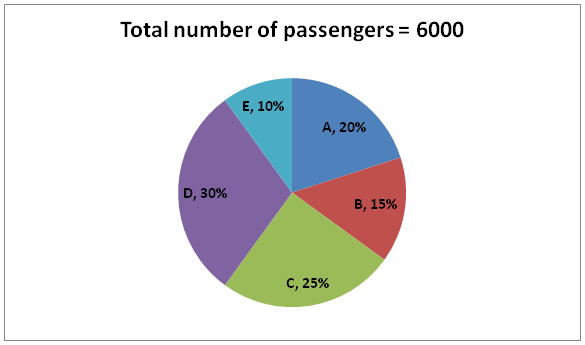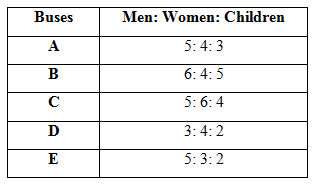# SBI Clerk Prelims 2021 Quantitative Aptitude Questions (Day-41)

Dear Aspirants, Our IBPS Guide team is providing new series of Quantitative Aptitude Questions for SBI Clerk Prelims 2020 so the aspirants can practice it on a daily basis. These questions are framed by our skilled experts after understanding your needs thoroughly. Aspirants can practice these new series questions daily to familiarize with the exact exam pattern and make your preparation effective.

Start Quiz

Missing number series

Directions (01-05): What value should come in the place of (?) in the following number series?

1) 12, 54, 124, 222, 348,?

A.498

B.486

C.502

D.512

E.490

2) 36, 54, 108, 270, 810, ?

A.3240

B.2835

C.1620

D.1215

E.2430

3) 678, 637, 594, 547, 494, ?

A.423

B.432

C.435

D.428

E.439

4) 24, 45, 80, 129, ?, 297

A.184

B.196

C.190

D.206

E.212

5) 23, 138, 690, ?, 8280, 16560

A.1840

B.2240

C.2760

D.3230

E.3890

Data Interpretation

Directions (06-10): Study the following information carefully and answer the questions given below.

The given pie chart shows the number of passengers in five buses – A, B, C, D and E.The given table shows the ratio of the number of Men, women and children passengers in the bus.6) The number of women passengers in bus A is what percent of the number of Men passengers in bus D?

A.44.45%

B.55.56%

C.66.67%

D.77.78%

E.33.33%

7) What is the average number of children passengers in all the buses together?

A.310

B.308

C.304

D.302

E.312

8) What is the ratio of the number of men to women passengers in B and C together?

A.40: 39

B.41: 40

C.42: 41

D.43: 42

E.None of these

9) What is the difference between the number of men and women passengers in all the buses together?

A.20

B.30

C.40

D.50

E.60

10) The number of men passengers in bus F is 20% more than the number of women passengers in bus A and the number of women passengers in F is 40% more than the number of men passengers in C. If the total number of passengers in F is 1300, then find the number of children passengers in F?

A.100

B.110

C.130

D.120

E.140

12 + (14 * 3) = 54

54 + (14 * 5) = 124

124 + (14 * 7) = 222

222 + (14 * 9) = 348

348 + (14 * 11) = 502

36 * 1.5 = 54

54 * 2 = 108

108 * 2.5 = 270

270 * 3 = 810

810 * 3.5 = 2835

678 – 41 = 637

637 – 43 = 594

594 – 47 = 547

547 – 53 = 494

494 – 59 = 435

24 + (3 * 7) = 45

45 + (5 * 7) = 80

80 + (7 * 7) = 129

129 + (11 * 7) = 206

206 + (13 * 7) = 297

23 * 6 = 138

138 * 5 = 690

690 * 4 = 2760

2760 * 3 = 8280

8280 * 2 = 16560

Directions (6-10):

Number of passengers in bus A = 20/100 * 6000 = 1200

Number of passengers in bus B = 15/100 * 6000 = 900

Number of passengers in bus C = 25/100 * 6000 = 1500

Number of passengers in bus D = 30/100 * 6000 = 1800

Number of passengers in bus E = 10/100 * 6000 = 600

Number of Men passengers in A = 5/12 * 1200 = 500

Number of women passengers in A = 4/12 * 1200 = 400

Number of children passengers in A = 3/12 * 1200 = 300

Number of Men passengers in B = 6/15 * 900 = 360

Number of women passengers in B = 4/15 * 900 = 240

Number of children passengers in B = 5/15 * 900 = 300

Number of Men passengers in C = 1500 * 5/15 = 500

Number of women passengers in C = 1500 * 6/15 = 600

Number of children passengers in C = 1500 * 4/15 = 400

Number of Men passengers in D = 3/9 * 1800 = 600

Number of women passengers in D = 4/9 * 1800 = 800

Number of children passengers in D = 2/9 * 1800 = 400

Number of Men passengers in E = 5/10 * 600 = 300

Number of women passengers in E = 3/10 * 600 = 180

Number of children passengers in E = 2/10 * 600 = 120

Required percentage = 400/600 * 100 = 66.67%

Required average = (300 + 300 + 400 + 400 + 120)/5

= 304

Required ratio = (360 + 500): (240 + 600)

= 860: 840

= 43: 42

Number of men passengers = (500 + 360 + 500 + 600 + 300) = 2260

Number of women passengers = (400 + 240 + 600 + 800 + 180) = 2220

Difference = 2260 – 2220 = 40

Total passengers in F = 1300

Number of men passengers in F = 120/100 * 400 = 480

Number of women passengers in F = 140/100 * 500 = 700

Number of children passengers in F = 1300 – 480 – 700 = 120

 Check Here to View SBI Clerk Prelims 2021 Quantitative Aptitude Questions Day – 40 Day – 39 Day – 38